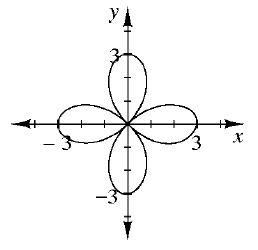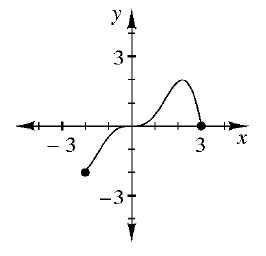### Home > INT3 > Chapter 4 > Lesson 4.2.2 > Problem4-59

4-59.

Examine each graph below. Decide if the graph represents a function and then state the domain and range.

1.For a relation to be a function, each input can only have one output. That is, each $x$-value can only have one corresponding $y$-value.

1.